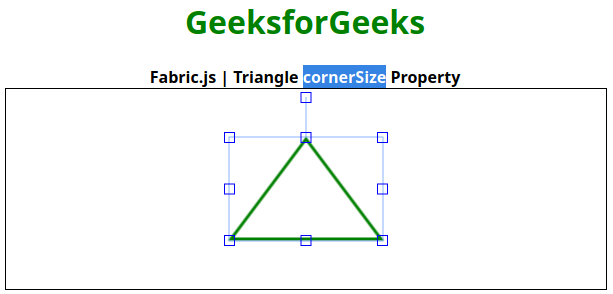# Fabric.js | Triangle cornerSize Property

• Last Updated : 09 Feb, 2021

In this article, we are going to see how to set the size of controlling corners of the canvas triangle using FabricJS. The canvas triangle means the triangle is movable and can be stretched according to requirement. Further, the triangle can be customized when it comes to initial stroke color, height, width, fill color, or stroke width.
To make this possible we are going to use a JavaScript library called FabricJS. After importing the library, we will create a canvas block in the body tag which will contain the triangle. After this, we will initialize instances of Canvas and triangle provided by FabricJS and set a corner size of controlling corners of the canvas triangle using cornerSize property and render the triangle on the Canvas as given in the below example.
Syntax:

```fabric.Triangle({
width: number,
height: number,
cornerSize: number
});```

Parameters: This function accepts three parameters as mentioned above and described below:

• width: It specifies the width of triangle.
• height: It specifies the height of triangle.
• cornerSize: This parameter defines the size of the controlling corners.

Example: This example uses FabricJS to set a size of controlling corners of the canvas-like triangle as given below. You have to click on the object to see the size of controlling corners.

## html

 ```<``html``>` `<``head``>``    ``<``title``>``        ``Fabric.js | Triangle cornerSize Property``    ````    ` `    ````    ``<``script` `src``=``"https://cdnjs.cloudflare.com/ajax/libs/fabric.js/3.6.2/fabric.min.js"``>``    ````` `<``body``>``        ``<``div` `style``=``"text-align: center;width: 600px;"``>``        ``<``h1` `style``=``"color: green;"``>``            ``GeeksforGeeks``        ````        ``<``b``>``            ``Fabric.js | Triangle cornerSize Property``        ````        ````    ``<``canvas` `id``=``"canvas"` `width``=``"600"` `height``=``"200"``        ``style``=``"border:1px solid #000000"``>``    ````    ` `    ``<``script``>``    ` `        ``// Initiate a Canvas instance``        ``var canvas = new fabric.Canvas("canvas");` `        ``// Initiate a triangle instance``        ``var triangle = new fabric.Triangle({``                        ``width: 150,``                        ``height: 100,``                        ``fill: '',``                        ``stroke: 'green',``                        ``strokeWidth: 3,``                        ``cornerColor: 'blue',``                        ``cornerSize: 10``        ``});` `        ``// Render the triangle in canvas``        ``canvas.add(triangle);``        ``canvas.centerObject(triangle);``    ````` `                   `

Output:My Personal Notes arrow_drop_up